## Sample Test Problems

1. Calculate the commutatorwhereand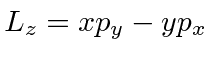. State the uncertainty principle for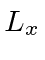and.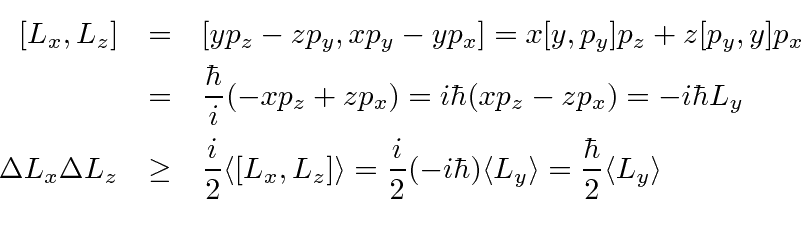2. * A particle of massis in a 1 dimensional potential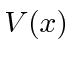. Calculate the rate of change of the expected values ofand, (and). Your answer will obviously depend on the state of the particle and on the potential.3. Compute the commutatorsandfor the 1D harmonic oscillator.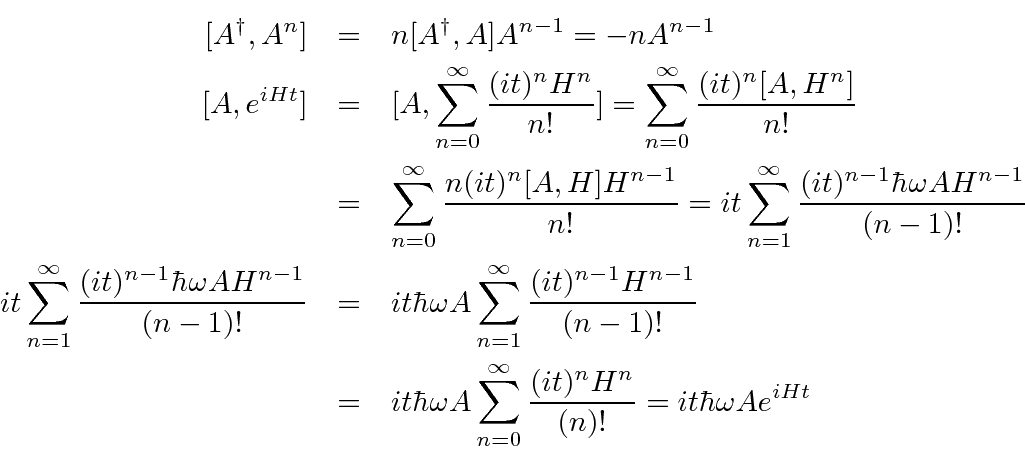4. * Assume that the statesare the eigenstates of the Hamiltonian with eigenvalues, ().
a)
Prove thatfor an arbitrary linear operator.
b)
For a particle of massmoving in 1-dimension, the Hamiltonian is given by. Compute the commutator [H,X] whereis the position operator.
c)
Computethe mean momentum in the state.
5. * At, a particle of massis in the Harmonic Oscillator state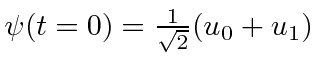. Use the Heisenberg picture to find the expected value ofas a function of time.

Jim Branson 2013-04-22